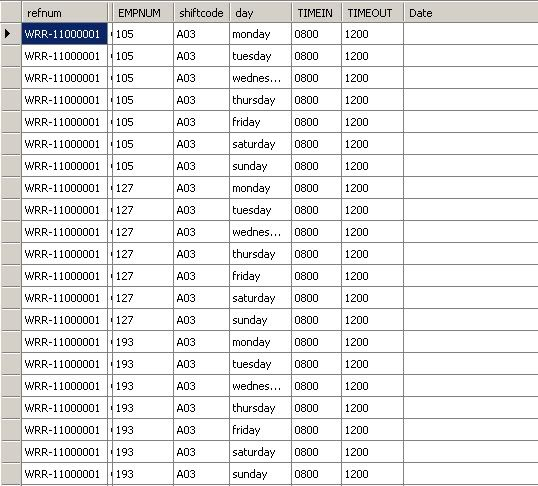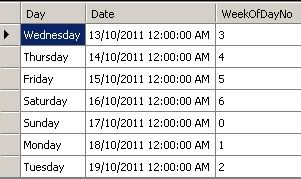#Update a column in a DataTable from a column belonging to a different DataTable in a DataSet• ### Question

•Guys,

I need help with this.  Basically I don't want to do it directly in the database.  I just want to do this in the memory.  As you can see below are pictures of

Employee Schedule DataTable

ESCHWeekly Date Schedule DataTable

WSCHSo what I hope to accomplish is how can I update the ESCH.DATE's column based on the the WSCH.DATE's column

Jason

Thursday, October 13, 2011 7:30 AM

•Something like:

```Dim qryWSCH As String
For Each drWSCH As DataRow in dtESCH.Rows
qryWSCH = "Day = '" & drWSCH("Day") & "'"

Dim rowsESCH() As DataRow = dtESCH.Select(qryWSCH)
For i = 0 To rowsESCH.Length - 1
Dim updRow as DataRow = rowsESCH(i)
updRow.BeginEdit()
updRow("Date") = drWSCH("Date")
Next i
Next
```

James Crandall ~ http://javitechnologies.com Spatial Database Solutions
Thursday, October 13, 2011 1:45 PM
•Here's a more complete example that I have tested.  eDT is the target DataTable to add the Date values to, wDT is the source table.

Hope this makes sense!

Good luck

```            Dim eDT As New DataTable("eDT")
Dim Ecol As DataColumn = New DataColumn()
Ecol.DataType = System.Type.GetType("System.String")
Ecol.ColumnName = "DayOfTheWeek"

Ecol = New DataColumn()
Ecol.DataType = System.Type.GetType("System.DateTime")
Ecol.ColumnName = "DateValue"

Dim newRow As DataRow = eDT.NewRow()
newRow("DayOfTheWeek") = "Monday"

newRow = eDT.NewRow()
newRow("DayOfTheWeek") = "Tuesday"

newRow = eDT.NewRow()
newRow("DayOfTheWeek") = "Saturday"

Dim wDT As New DataTable("wDT")
Dim Wcol As DataColumn = New DataColumn()
Wcol.DataType = System.Type.GetType("System.String")
Wcol.ColumnName = "DayOfTheWeek"

Wcol = New DataColumn()
Wcol.DataType = System.Type.GetType("System.DateTime")
Wcol.ColumnName = "DateValue"

newRow = wDT.NewRow()
newRow("DayOfTheWeek") = "Monday"
newRow("DateValue") = "5/5/2011"

wDT.AcceptChanges()

newRow = wDT.NewRow()
newRow("DayOfTheWeek") = "Tuesday"
newRow("DateValue") = "7/1/2011"

wDT.AcceptChanges()

newRow = wDT.NewRow()
newRow("DayOfTheWeek") = "Saturday"
newRow("DateValue") = "10/5/2011"

wDT.AcceptChanges()

Dim counti As Integer = wDT.Rows.Count

Dim dayVal As String
Dim dateVal As DateTime

For Each dr As DataRow In eDT.Rows
dayVal = dr("DayOfTheWeek")
If Not IsDBNull(dr("DateValue")) Then
dateVal = dr("DateValue")
End If
Next

For Each wdr As DataRow In wDT.Rows
dayVal = wdr("DayOfTheWeek")
If Not IsDBNull(wdr("DateValue")) Then
dateVal = wdr("DateValue")
End If
Next

Dim qryWSCH As String
For Each drWSCH As DataRow In wDT.Rows
qryWSCH = "DayOfTheWeek = '" & drWSCH("DayOfTheWeek") & "'"
dateVal = drWSCH("DateValue")
Dim rowsESCH() As DataRow = eDT.Select(qryWSCH)
For i = 0 To rowsESCH.Length - 1
Dim updRow As DataRow = rowsESCH(i)
updRow.BeginEdit()
updRow("DateValue") = drWSCH("DateValue")
updRow.EndEdit()
eDT.AcceptChanges()
Next i
Next

For Each dr As DataRow In eDT.Rows
dayVal = dr("DayOfTheWeek")
dateVal = dr("DateValue")
Next
```

James Crandall ~ http://javitechnologies.com Spatial Database Solutions
Thursday, October 13, 2011 4:34 PM

### All replies

•Something like:

```Dim qryWSCH As String
For Each drWSCH As DataRow in dtESCH.Rows
qryWSCH = "Day = '" & drWSCH("Day") & "'"

Dim rowsESCH() As DataRow = dtESCH.Select(qryWSCH)
For i = 0 To rowsESCH.Length - 1
Dim updRow as DataRow = rowsESCH(i)
updRow.BeginEdit()
updRow("Date") = drWSCH("Date")
Next i
Next
```

James Crandall ~ http://javitechnologies.com Spatial Database Solutions
Thursday, October 13, 2011 1:45 PM
•Here's a more complete example that I have tested.  eDT is the target DataTable to add the Date values to, wDT is the source table.

Hope this makes sense!

Good luck

```            Dim eDT As New DataTable("eDT")
Dim Ecol As DataColumn = New DataColumn()
Ecol.DataType = System.Type.GetType("System.String")
Ecol.ColumnName = "DayOfTheWeek"

Ecol = New DataColumn()
Ecol.DataType = System.Type.GetType("System.DateTime")
Ecol.ColumnName = "DateValue"

Dim newRow As DataRow = eDT.NewRow()
newRow("DayOfTheWeek") = "Monday"

newRow = eDT.NewRow()
newRow("DayOfTheWeek") = "Tuesday"

newRow = eDT.NewRow()
newRow("DayOfTheWeek") = "Saturday"

Dim wDT As New DataTable("wDT")
Dim Wcol As DataColumn = New DataColumn()
Wcol.DataType = System.Type.GetType("System.String")
Wcol.ColumnName = "DayOfTheWeek"

Wcol = New DataColumn()
Wcol.DataType = System.Type.GetType("System.DateTime")
Wcol.ColumnName = "DateValue"

newRow = wDT.NewRow()
newRow("DayOfTheWeek") = "Monday"
newRow("DateValue") = "5/5/2011"

wDT.AcceptChanges()

newRow = wDT.NewRow()
newRow("DayOfTheWeek") = "Tuesday"
newRow("DateValue") = "7/1/2011"

wDT.AcceptChanges()

newRow = wDT.NewRow()
newRow("DayOfTheWeek") = "Saturday"
newRow("DateValue") = "10/5/2011"

wDT.AcceptChanges()

Dim counti As Integer = wDT.Rows.Count

Dim dayVal As String
Dim dateVal As DateTime

For Each dr As DataRow In eDT.Rows
dayVal = dr("DayOfTheWeek")
If Not IsDBNull(dr("DateValue")) Then
dateVal = dr("DateValue")
End If
Next

For Each wdr As DataRow In wDT.Rows
dayVal = wdr("DayOfTheWeek")
If Not IsDBNull(wdr("DateValue")) Then
dateVal = wdr("DateValue")
End If
Next

Dim qryWSCH As String
For Each drWSCH As DataRow In wDT.Rows
qryWSCH = "DayOfTheWeek = '" & drWSCH("DayOfTheWeek") & "'"
dateVal = drWSCH("DateValue")
Dim rowsESCH() As DataRow = eDT.Select(qryWSCH)
For i = 0 To rowsESCH.Length - 1
Dim updRow As DataRow = rowsESCH(i)
updRow.BeginEdit()
updRow("DateValue") = drWSCH("DateValue")
updRow.EndEdit()
eDT.AcceptChanges()
Next i
Next

For Each dr As DataRow In eDT.Rows
dayVal = dr("DayOfTheWeek")
dateVal = dr("DateValue")
Next
```

James Crandall ~ http://javitechnologies.com Spatial Database Solutions
Thursday, October 13, 2011 4:34 PM
••